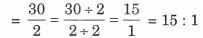# NCERT Solutions for Class 6 Maths Chapter 12 Ratios and Proportions Ex 12.1

ncert textbook

## NCERT Solutions for Class 6 Maths Chapter 12 Ratios and Proportions Ex 12.1

NCERT Solutions For Class 6 Maths Chapter 12 Ratios and Proportions Ex 12.1

Exercise 12.1

Ex 12.1 Class 6 Maths Question 1.
There are 20 girls and 15 boys in a class.
(a) What is the ratio of the number of girls to the number of boys?
(b) What is the ratio of the number of girls to the number of students in the class?
Solution:
(a) Number of girls = 20
Number of boys = 15
Total number of students = 20 + 15 = 35
∴ Ratio of the number of girls to the number of boys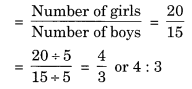Thus, the required ratio is 4 : 3.
(b) Ratio of the number of girls to the number of studentsThus, the required ratio is 4 : 7.

Ex 12.1 Class 6 Maths Question 2.
Out of 30 students in a class, 6 like football, 12 like cricket and remaining like tennis. Find the ratio of
(a) Number of students liking football to the number of students liking tennis.

(b) Number of students liking cricket to total number of students.Solution:
Number of students in the class = 30
Number of students liking football = 6
Number of students liking cricket = 12
Number of students liking tennis = 30 – (6 + 12) = 30 – 18 = 12

(a) Ratio of the number of the students liking football to the number of students liking tennisThus, the required ratio is 1 : 2.

(b) Ratio of the number of students liking cricket to the total number of studentsThus, the required ratio is 2 : 5.

Ex 12.1 Class 6 Maths Question 3.
See the figure and find the ratio of
(а) Number of triangles to the number of circles inside the rectangle.
(b) Number of squares to all the figures inside the rectangle.
(c) Number of circles to all the figures inside the rectangle.Solution:
(a) Number of triangles 3
Number of circles = 2
∴ Ratio of number of triangles to the number of circles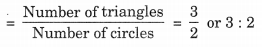Thus, the required ratio is 3 : 2.

(b) Number of squares = 2
Number of all figures = 7
∴ Ratio of number of squares to the number of all the figuresThus, the required ratio is 2 : 7.

(c) Ratio of number of circles to the number of all the figuresThus, the required ratio is 2 : 7.

Ex 12.1 Class 6 Maths Question 4.
Distances travelled by Hamid and Akhtar in an hour are 9 km and 12 km. Find the ratio of speed of Hamid to the speed of Akhtar.
Solution:
Distance travelled by Hamid = 9 km.
Distance travelled by Akhtar = 12 km.
Speed of Hamid = 9 km
per hour Speed of Akhtar = 12 km per hour
∴ Ratio of the speed of Hamid to the speed of Speed of Hamid ar = Speed of AkhtarThus, the required ratio is 3 : 4.

Ex 12.1 Class 6 Maths Question 5.
Fill in the following blanks: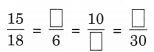[Are these equivalent ratios?]
Solution: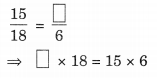Now the fractions, we haveEx 12.1 Class 6 Maths Question 6.
Find the ratio of the following:
(a) 81 to 108
(b) 98 to 63
(c) 33 km to 121 km
(d) 30 minutes to 45 minutes
Solution:Ex 12.1 Class 6 Maths Question 7.
Find the ratio of the following:
(a) 30 minutes to 1.5 hours
(b) 40 cm to 1.5 m
(c) 55 paise to ₹ 1
(d) 500 mL to 2 litres
Solution:
(a) 1 hour = 60 minutes
∴ 1.5 hours = 60 x 1.5 minutes = 90 minutes
∴ Ratio of 30 minutes to 1.5 hours = Ratio of 30 minutes to 90 minutes(b) 1 m = 100 cm
∴ 1.5 m = 1.5 x 100 cm = 150 cm.
∴ Ratio of 40 cm to 1.5 m = Ratio of 40 cm to 150 cm.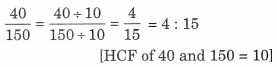(c) ₹1 = 100 paise
∴ Ratio of 55 paise to ₹ 1 = Ratio of 55 paise to 100 paise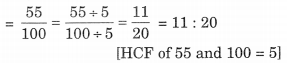(d) 500 mL to 2 litres
1 litre = 1000 mL
∴ 2 litres = 2 x 1000 mL = 2000 mL
∴ Ratio of 500 mL to 2 litres = Ratio of 500 mL to 2000 mLEx 12.1 Class 6 Maths Question 8.
In a year, Seema earns ₹ 1,50,000 and saves ₹ 50,000. Find the ratio of
(a) Money that Seema earns to the money she saves.
(b) Money that she saves to the money she spends.
Solution:
(a) Money earned by Seema = ₹ 1,50,000
Money saved by her = ₹ 50,000
∴ Money spent by her = ₹ 1,50,000 – ₹ 50,000 = ₹ 1,00,000
∴ Ratio of money earned by Seema to the money saved by her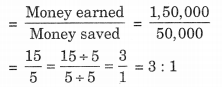(b) Ratio of money saved by Seema to the moneyEx 12.1 Class 6 Maths Question 9.
There are 102 teachers in a school of 3300 students. Find the ratio of the number of teachers to the number of students.
Solution:
Number of teachers = 102
Number of students = 3300
∴ Ratio of number of teachers to the number of studentsEx 12.1 Class 6 Maths Question 10.
In a college, out of 4320 students, 2300 are girls, find the ratio of
(а) Number of girls to the total number of students.
(b) Number of boys to the number of girls.
(c) Number of boys to the total number of students.
Solution:
Total number of students = 4320
Number of girls = 2300
∴ Number of boys = 4320 – 2300 = 2020

(a) Ratio of number of girls to the total number of students(b) Ratio of number of boys to the number of girls(c) Ratio of number of boys to the total number of students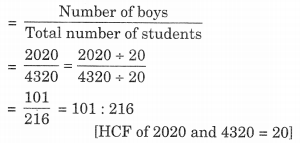Ex 12.1 Class 6 Maths Question 11.
Out of 1800 students in a school, 750 opted basketball, 800 opted cricket and remaining opted table tennis. If a student can opt only one game, find the ratio of
(а) Number of students who opted basketball to the number of students who opted table tennis.
(b) Number of students who opted cricket to the number of students opting basketball.
(c) Number of students who opted basketball to the total number of students.
Solution:
Total number of students = 1800
Number of students opting basketball = 750
Number of students who opted cricket = 800
Number of remaining students who opted table tennis = 1800 – (750 + 800)
= 1800 – 1550 = 250

(а) Ratio of number of students opted basketball to the number of students who opted table tennis
Number of students opting basketball Number of students opting table tennis(b) Ratio of the students who opted cricket to the number of students opting basketball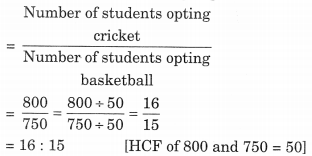(c) Ratio of number of students who opted basketball to the total number of studentsEx 12.1 Class 6 Maths Question 12.
Cost of a dozen pens is ₹180 and cost of 8 ball pens is ₹56. Find the ratio of the cost of a pen to the cost of a ball pen.
Solution:
Cost of 1 dozen, i.e., 12 pens = ₹180
∴ Cost of 1 pen = ₹$\frac { 180 }{ 12 }$= ₹15
Cost of 8 ball pens = ₹56
∴ Cost of 1 ball pen = ₹$\frac { 56 }{ 8 }$= ₹ 7
Ratio of cost of 1 pen to cost of 1 ball penThus required ratio is 15 : 7.

Ex 12.1 Class 6 Maths Question 13.
Consider the statement : Ratio of breadth and length of a hall is 2 : 5. Complete the following table that shows some possible breadths and lengths of the hall.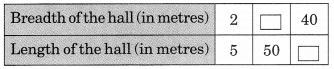Solution: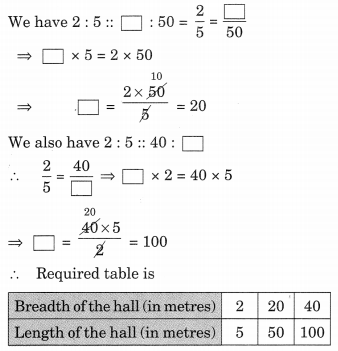Ex 12.1 Class 6 Maths Question 14.
Divide 20 pens between Sheela and Sangeeta in the ratio of 3 : 2.
Solution:
We have 3 + 2 = 5
Total number of pen = 20
∴ Sheela’s share =$\frac { 3 }{ 5 }$x 20 = 3 x 4 = 12 pens 5
Sangeeta’s shares =$\frac { 2 }{ 5 }$x 20 = 2 x 4 = 8 pens.
Thus Sheela gets 12 pens and Sangeeta gets 8 pens.

Ex 12.1 Class 6 Maths Question 15.
Mother wants to divide ₹ 36 between her daughters Shreya and Bhoomika in the ratio of their ages. If age of Shreya is 15 years and age of Bhoomika is 12 years, find how much Shreya and Bhoomika will get?
Solution:
Given that:
Money got by Shreya : Money got by Bhoomika = 15 : 12
∴ Sum = 15 + 12 = 27Ex 12.1 Class 6 Maths Question 16.
Present age of father is 42 years and that of his son is 14 years. Find the ratio of
(a) Present age of father to the present age of son.
(b) Age of the father to the age of son, when son was 12 years old.
(c) Age of father after 10 years to the age of son after 10 years.
(d) Age of father to the age of son when father was 30 years old.
Solution:
Present age of father = 42 years.
Present age of his son = 14 years.
(a) Ratio of present age of father to the present age of son(b) When son was 12 years old, i.e., 14 – 12 = 2 years ago father’s age = 42 – 2 = 40 years.
Ratio of the father’s age to the son’s age(c) Ratio of father’s age after 10 years, i.e., 42 + 10 = 52 years
to the age of son after 10 years, i.e., = 14 + 10 = 24 years(d) Ratio of the son’s age to the age of father when he was only 30 years .
When father was 30 years,
i.e., before 42 – 30 = 12 years
Age of son was = 14 – 12 = 2 years
∴ Required ratio